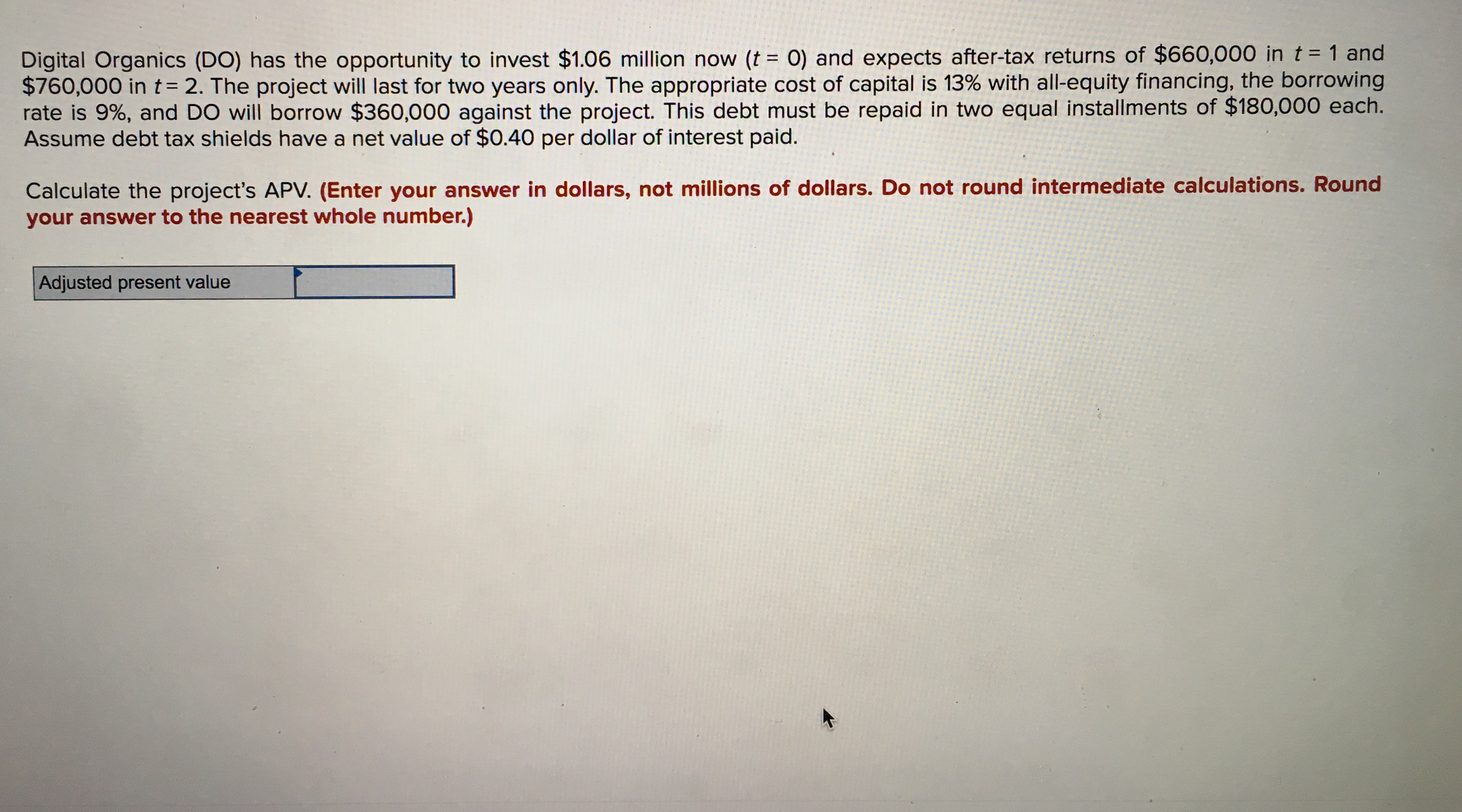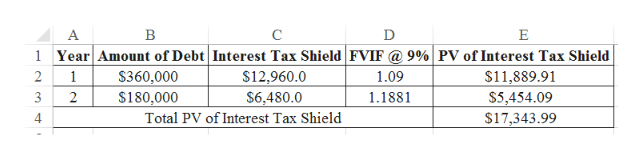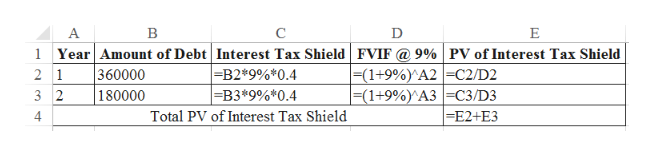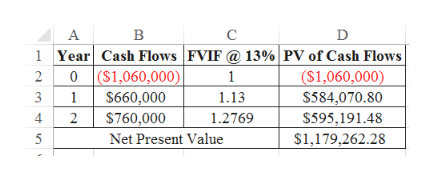Digital Organics (DO) has the opportunity to invest \$1.06 million now (t = 0) and expects after-tax returns of \$660,000 in t = 1 and\$760,000 in t= 2. The project will last for two years only. The appropriate cost of capital is 13% with all-equity financing, the borrowingrate is 9%, and DO will borrow \$360,000 against the project. This debt must be repaid in two equal installments of \$180,000 each.Assume debt tax shields have a net value of \$0.40 per dollar of interest paid.Calculate the project's APV. (Enter your answer in dollars, not millions of dollars. Do not round intermediate calculations. Roundyour answer to the nearest whole number.)Adjusted present value

Questionhelp_outlineImage TranscriptioncloseDigital Organics (DO) has the opportunity to invest \$1.06 million now (t = 0) and expects after-tax returns of \$660,000 in t = 1 and \$760,000 in t= 2. The project will last for two years only. The appropriate cost of capital is 13% with all-equity financing, the borrowing rate is 9%, and DO will borrow \$360,000 against the project. This debt must be repaid in two equal installments of \$180,000 each. Assume debt tax shields have a net value of \$0.40 per dollar of interest paid. Calculate the project's APV. (Enter your answer in dollars, not millions of dollars. Do not round intermediate calculations. Round your answer to the nearest whole number.) Adjusted present value fullscreen
Step 1

Calculation of Total PV of Interest Tax Shield:help_outlineImage TranscriptioncloseC E YearAmount of Debt Interest Tax Shield FVIF @ 9%| PV of Interest Tax Shield 1 \$360,000 S180,000 \$11,889.91 \$5,454.09 \$17,343.99 2 1 \$12,960.0 1.09 1.1881 3 2 \$6,480.0 4 Total PV of Interest Tax Shield fullscreen
Step 2

Excel Workings:help_outlineImage TranscriptioncloseA C E Year Amount of Debt Interest Tax Shield FVIF @ 9% | PV of Interest Tax Shield 360000 180000 |=(1+9%)^A2 | =C2/D2 |=(1+9%)^A3 | =C3/D3 =E2+ E3 |=B2*9%*0.4 =B3*9%*0.4 2 1 3 2 4 Total PV of Interest Tax Shield fullscreen
Step 3

Calculation of NPV:

...help_outlineImage TranscriptioncloseD 1 Year Cash Flows FVIF @ 13%|PV of Cash Flows (\$1,060,000) \$584,070.80 \$595,191.48 0 (S1,060,000) \$660,000 \$760,000 Net Present Value 2 1 1 1.13 4 2 1.2769 5 s1,179,262.28 fullscreen

Want to see the full answer?

See Solution

Want to see this answer and more?

Our solutions are written by experts, many with advanced degrees, and available 24/7

See Solution
Tagged in